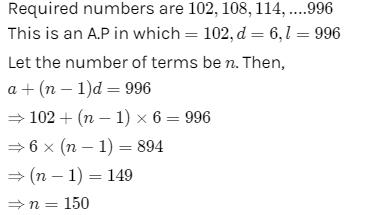Courses

# Test: Algebra - 1

## 10 Questions MCQ Test Mathematics (Maths) Class 6 | Test: Algebra - 1

Description
This mock test of Test: Algebra - 1 for Class 6 helps you for every Class 6 entrance exam. This contains 10 Multiple Choice Questions for Class 6 Test: Algebra - 1 (mcq) to study with solutions a complete question bank. The solved questions answers in this Test: Algebra - 1 quiz give you a good mix of easy questions and tough questions. Class 6 students definitely take this Test: Algebra - 1 exercise for a better result in the exam. You can find other Test: Algebra - 1 extra questions, long questions & short questions for Class 6 on EduRev as well by searching above.
QUESTION: 1

Solution:
QUESTION: 2

Solution:
QUESTION: 3

### Number of matchsticks required to make a pattern of “E”

Solution:

Number of matchsticks required to make one= 5
∴ Number of matchsticks required to make a pattern of letter E as= 5n

QUESTION: 4

A basket has x mangoes, how many mangoes are there in 5 baskets?

Solution:
QUESTION: 5

Perimeter of the square, whose each side is ‘n’ cm is

Solution:
QUESTION: 6

Diameter of circle whose radius is ‘r’ is

Solution:

Diameter is twice the radius, so in this case it'll be 2r

QUESTION: 7

The number of 3-digit numbers divisible by 6, is …………...

Solution:QUESTION: 8

The side of an equilateral triangle is shown by l. Express the perimeter of the equilateral triangle using l.

Solution:
QUESTION: 9

Give expression for 25 added to r.

Solution:
QUESTION: 10

Which out of the following are expressions with numbers only?

Solution: